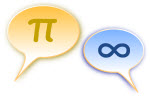# The Language of Mathematics

## The Language of Mathematics

The Language of Mathematics was designed so we can write about:

Things like Numbers, Sets, Functions, etc

What we Do with those things (add, subtract, multiply, divide, join together, etc)

## SymbolsMathematics uses symbols instead of words:

• There are the 10 digits: 0, 1, 2, ... 9
• There are symbols for operations: +, −, ×, /, ...
• And symbols that "stand in" for values: x, y, ...
• And many special symbols: π, =, <, ≤, ...

## Letter Conventions

Letters often have special uses:

Examples What they usually mean
Start of the alphabet: a, b, c, ... constants (fixed values)
From i to n: i, j, k, l, m, n positive integers (for counting)
End of the alphabet: ... x, y, z variables (unknowns)

Those are not rules, but they are often used that way.

### Example: y = ax + b

People will assume that a and b are fixed values,

And that x is the one that changes, which in turn makes y change.

## UPPERCASE vs lowercase

It is also common to use

• lowercase for variables (like x or y) or counting values (like m or n) and
• UPPERCASE for sets (like X or Y) and special constants

### Example: A = {1, 2, 3}

Using an uppercase "A" makes it easy to tell it is a set.

It makes things clearer to read.

## Nouns, Verbs, Sentences

We don't use the words "noun", "verb", or "pronoun" in Mathematics, but we can imagine these similarities to English:

Nouns could be fixed things, such as numbers, or expressions with numbers:
 15 2(3-1/2) 42
The Verb could be the equals sign "=", or an inequality like < or >
Pronouns (things like it, he, you, etc) could be variables like x or y:
 5x-7 xy2 -3/x
An Adjective could be a subscript like the "n" in xn
And they could be put together into a Sentence like this:

3x + 7 = 22

(And we actually do use the word sentence in mathematics!)